# Lesson Notes By Weeks and Term - Primary 3

Multiplication

TERM: 2nd Term

WEEK: 1

CLASS: Primary 3

AGE: 8 years

DURATION: 5 periods of 40 minutes each

DATE:

SUBJECT: Mathematics

TOPIC: Multiplication

SPECIFIC OBJECTIVES: At the end of the lesson, the pupils should be able to

1. Learn multiplication tables 1, 8 and 9 times table

INSTRUCTIONAL TECHNIQUES: Explanation, question and answer, demonstration, practical, assessments

INSTRUCTIONAL MATERIALS: Multiplication tables, enlarged multiplication tables for the teacher, array diagram, enlarged array diagram, multiplication cards, videos from source-

PERIOD 1: The 9 times table

PRESENTATION

TEACHER’S ACTIVITY

PUPIL’S ACTIVITY

STEP 1

MENTAL MATHS

The teacher begins the lesson with some mental calculations

Calculate

a. 18 + 9 =

b. 27 + 9 =

c. 36 + 9 =

d. 0 + 9 =

e. 9 + 9 =

f. 54 + 9 =

g. 63 + 9 =

h. 45 + 9 =

i. 72 + 9 =

j. 81 + 9 =

Pupils respond and participate

STEP 2

CONCEPT

DEVELOPMENT

The teacher gives each pair of learners an array diagram and writes the following multiplication number sentence on the board:What do you notice about the answers that you recorded in your classwork book? (Learners may say that they see that the answers increase by 9 each time.)

NOTE: You may need to show learners the array diagrams for each of the calculations (as shown below) to help learners see that each time there is a row of 9 added.

In pairs,

The teacher asks learners to use their array diagrams to find the answers to the following:

5 × 9

6 × 9

7 × 9

8 × 9

9 × 9

Learners need to record the number sentences and answers in their classwork books.

Learners can then use these calculations and answers to create the rest of the 9 times table (up to 9 × 9).

The teacher draws this table on the board:

The table is completed together as a class.

 1 2 3 4 5 6 7 8 9 x 9 9 18 27 36 45 54 63 72 81

The learners are also asked to represent the table in a flowchart diagram

Pupils pay attention and participate

STEP 3

CLASS-WORK

Each pair or each group of learners is given a set of multiplication cards

for the 9 times table. Learners need to prepare these cards (cut them up and write the answers on the back) as they did for the multiplication cards of the other times tables in previous lessons. There are 4 activities suggested below – you can do all of them if you have enough time, otherwise select 2 or 3 activities to do in this lesson.

Rules of the game

1 Learners work in pairs.

a Learners shuffle the cards.

b One learner holds up a number sentence for the second learner to read.

c The second learner must read the number sentence and give the answer.

d The second learner then holds up a number sentence card for the first learner.

e Keep going until all the cards have been read.

2 Learners work in pairs.

a Learners shuffle the cards.

b Learners each hold up a number sentence card.

c Learners need to say the answer to each other’s cards.

d Learners then each select another number sentence card to hold up.

3 Learners work in groups of 3.

a Learners shuffle the cards.

b Learners lay out the cards with the answers facing up.

c One learner calls out a multiplication number sentence.

d The other two learners need to find the card with the answer to the

multiplication number sentence.

e The first learner to find the correct card gets to keep the card.

f When there are no more cards left, the game can be played again with a

different learner calling out the multiplication number sentences.

4 Learners work in pairs.

a Learners shuffle the cards.

b Learners lay out the cards with the answers facing up.

c One learner selects a card.

d The other learner must look at the answer and give a multiplication number

e If the learner says the correct multiplication number sentence then they can

keep the card.

f The second learner then selects a card for the first learner..

Pupils attempt their class work

STEP 4

HOME-WORK

Complete the table

 Multiplication Repeated addition Answer 1 5 x 9 9 + 9 + 9 + 9 + 9 2 3 x 9 3 9 + 9 + 9 + 9 + 9 + 9 + 9 4 2 x 9 5 9 + 9 + 9 + 9 + 9 + 9 + 9 + 9 + 9

Pupils attempt their class work

STEP 5

SUMMARY

The teacher summarizes by reminding the pupils how to solve multiplication tables up to the 9 times table.

She marks their class works, makes corrections where necessary and commends them positively

PERIOD 2: The 8 and 9 times table

 PRESENTATION TEACHER’S ACTIVITY PUPIL’S ACTIVITY STEP 1MENTAL MATHS The teacher begins the lesson with some mental calculations Calculate1. 8 x 2 =2. 8 x 1 =3. 8 x 3 =4. 8 x 5 =5. 8 x 4 =6. 9 x 3 =7. 9 x 2 =8. 9 x 1 =9. 9 x 5 =10. 9 x 4 = Pupils respond and participate STEP 2CONCEPTDEVELOPMENT The teacher* Gives the learners array diagrams to work with.• Writes the following problem on the chalkboard: There are 6 whole pizzas.Each pizza is cut into 8 pieces.How many pieces of pizza are there in total?NOTE: The word problem must be written on three lines as shown above to assist learners toidentify the critical information/numbers needed to solve the problem.• Reads the problem.• Lets the learners read the problem until they read it fluently.• Underlines the numbers (6 and 8).• Underlines the question (How many pieces of pizza are there in total?) with a wavy line.• Lets learners work out the answer using their array diagrams.• Lets learners present their ideas about how to solve the problem.• Asks learners to write the number sentence:6 × 8 = 48• Reads the number sentence together several times, making the connection between the numbers in the number sentence and the story of the word problem.• Confirms that the answer is 48 pieces of pizza. The teacher* Gives the learners array diagrams to work with.• Writes the following problem on the chalkboard: There are 7 bagsof 9 oranges each.How many oranges are there in total? • Reads the problem.• Lets the learners read the problem until they read it fluently.• Underlines the numbers (7 and 9).• Underlines the question (How many oranges are there in total?) with a wavy line.• Lets learners work out the answer using their array diagrams.• Lets learners present their ideas about how to solve the problem.• Asks learners to write the number sentence:7 × 9 = 63• Reads the number sentence together several times, making the connection between the numbers in the number sentence and the story of the word problem.• Confirms that the answer is 63 oranges. Pupils pay attention and participate STEP 3CLASS-WORK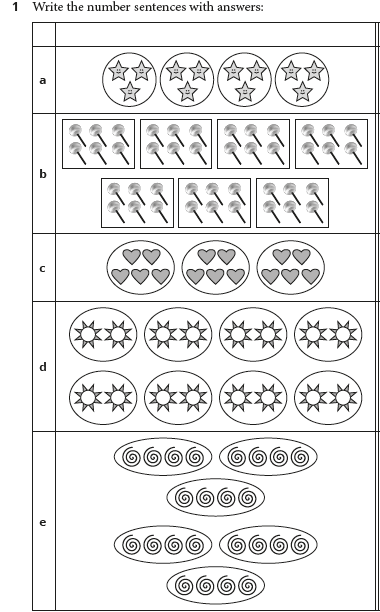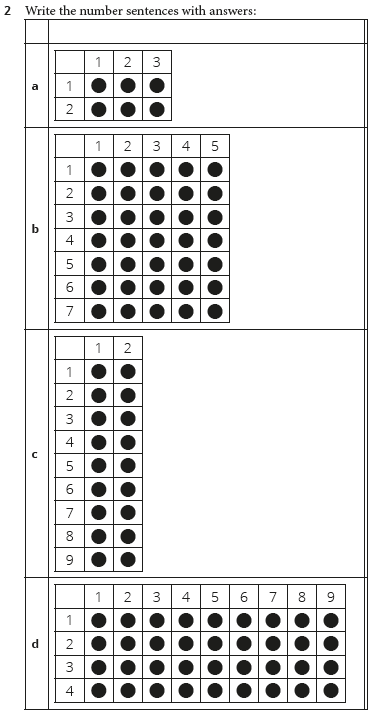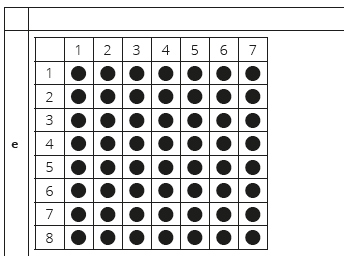Pupils attempt their class work STEP 4HOME-WORK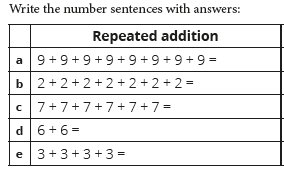Pupils attempt their class work STEP 5SUMMARY The teacher summarizes by reminding the pupils how to solve 8 and 9 times table.  She marks their class works, makes corrections where necessary and commends them positively

PERIOD 3: The 1 times table

PRESENTATION

TEACHER’S ACTIVITY

PUPIL’S ACTIVITY

STEP 1

MENTAL MATHS

The teacher begins the lesson with some mental calculations

Count forwards in 2s up to 20, in 3s up to 30, in 4s up to 40 and in 5s up to 50

Pupils respond and participate

STEP 2

CONCEPT

DEVELOPMENT

The teacher

Writes the following problem on the board:

There are 5 bags of fruit.

Each bag contains 2 oranges

and 1 apple.

NOTE: The word problem must be written on three lines as shown above to assist learners to

identify the critical information/numbers needed to solve the problem.

Underlines the numbers that tell us about the fruit (5, 2, 1).

• Asks: How many oranges are there in total?

• Asks: How many apples are there in total?

• Asks learners to write the number sentence for both fruits.

5 × 2 = 10 oranges

5 × 1 = 5 apples

• Asks: Why do both number sentences start with 5 × ? (This is a VERY important

discussion. Because there are 5 bags of fruit, each with two different types of fruit.)

• Confirms that the answer is 10 oranges and 5 apples.

CLASS ACTIVITY

The teacher

* Give each pair of learners an array diagram.

• Asks: How do we show 4 × 1 = using an array? (Allow learners time to think

• Shows the class the array diagrams (as shown below) which indicate how the answer to 4 ×

1 can be shown using an array.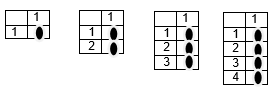Asks: What is 4 × 1? (Learners could hold up their fingers to show the answer.)

Repeats with all the 1 times table number sentences (in a random order) up to 9 × 1.

(The answer is the same as the number of groups).

Uses this as an opportunity to see whether learners have identified the rule of the 1 times table.

CLASS ACTIVITY II

Give each learner, each pair or each group of learners a set of multiplication cards for the 1 times table. Learners should have prepared these cards in the previous lesson.

Rules of the game

1 Learners work in pairs.

a Learners shuffle the cards.

b Learners each hold up a number sentence card.

c Learners need to say the answer to each other’s cards.

d Learners then each select another number sentence card to hold up.

2 Learners work in pairs.

a Learners shuffle the cards.

b Learners lay out the cards with the answers facing up.

c One learner selects a card.

d The other learner must look at the answer and say the correct multiplication

number sentence.

e If the learner says the correct multiplication number sentence they can keep

the card.

f The second learner then selects a card for the first learner.

Pupils pay attention and participate

STEP 3

CLASS-WORK

1. Write the number sentences with answers

 Number sentence 1 9 groups of 1 2 7 groups of 4 3 5 groups of 1 4 6 groups of 3 5 1 group of 1

2. Calculate

a. 4 x 1 =

b. 2 x 8 =

c. 8 x 1 =

d. 3 x 7 =

e. 4 x 6 =

f. 5 x 8 =

g. 6 x 7 =

h. 8 x 8 =

3.

 Repeated addition Number sentence 1 4 + 4 + 4 + 4 = 2 6 + 6 + 6 + 6 + 6 + 6 + 6 + 6 + 6 = 3 8 + 8 + 8 + 8 + 8 = 4 3 + 3 + 3 + 3 + 3 + 3 + 3 = 5 5 + 5 + 5 + 5 + 5 =

Pupils attempt their class work

STEP 4

HOME-WORK

Write the number sentences with answers

 Number sentence 1 3 groups of 1 2 4 groups of 6 3 8 groups of 1 4 2 groups of 7 5 5 groups 1

Pupils attempt their class work

STEP 5

SUMMARY

The teacher summarizes by reminding the pupils the rules for the 1 times table and times table up to 9.

She marks their class works, makes corrections where necessary and commends them positively

PERIOD 4: Doubling and repeated addition

PRESENTATION

TEACHER’S ACTIVITY

PUPIL’S ACTIVITY

STEP 1

MENTAL MATHS

The teacher begins the lesson with some mental calculations

Calculate

a. 2 x 3 =

b. 2 x 1 =

c. 2 x 4 =

d. 2 x 2 =

e. 2 x 5 =

f. 2 x 6 =

g. 2 x 8 =

h. 2 x 9 =

i. 2 x 7 =

j. 2 x 10 =

Pupils respond and participate

STEP 2

CONCEPT

DEVELOPMENT

The teacher

* Calls out a number sentence to the class, without giving the answer:

• 2 × 10 = ?

• Asks learners solve the problem as quickly as possible and tell their partner the answer.

Allows learners to write in their classwork books if they need to work out the answer, however the goal is for learners to be able to solve these problems mentally by doubling or saying two tens is 20.

Asks: How did you solve this problem? (2 groups of 10, I doubled 10.)

Takes some time to discuss the fact that 10 + 10 is the same as 2 × 10.

Repeats with different problems involving doubles of the multiples of 5 up to 50. ( i.e. 2 ×

(multiples of 5 up to 50)):

Asks the learners to calculate the following:

2 × 20

2 × 15

2 × 5

2 × 30

2 × 25

2 × 40

2 × 35

2 × 50

2 × 45

CLASS ACTIVITY

The teacher

Writes the following problem on the board:

On one day at the clinic 45 learners were given flu vaccinations.

The next day 46 learners were vaccinated.

How many learners were vaccinated altogether?

NOTE: The word problem must be written on three lines as shown above to assist learners to

identify the critical information/numbers needed to solve the problem.

Underlines the numbers (45, 46).

Underlines the question (How many learners were vaccinated altogether?) with a

wavy line.

Lets learners work out the answer. (45 + 46 = )

Lets learners present their ideas about how to solve the problem.

The focus here is on getting learners to see that they can solve the problem through doubling. Observe learners as they solve the problem, and select different learners to present their solutions. Ensure that at least one of the selected learners solved the problem by doubling. If you can’t identify any learners solving the problem through doubling, then you will need to model doubling as a strategy yourself.

Asks a learner to show their solution on the board:

45 + 45 + 1 = 91

2 × 45 = 90

90 + 1 = 91 learners were vaccinated

Another way of finding the answer to this problem through doubling may be:

46 + 46 – 1 = 91

2 × 46 = 92

92 – 1 = 91 learners were vaccinated

Confirms that the answer is 91 learners.

ACTIVITY II

The teacher

Draws the following table on the board:

 1 2 3 4 5 6 7 8 9 x 5 x 10

Asks learners to come up to the board to complete the answers to the × 5 row and then

the × 10 row. (Call up many different learners to help complete the table so that the whole class participates in the activity.)

Asks: What do you notice about the numbers we filled in for the × 5 and the × 10

rows? (Discuss all the observations. They all end in 5 or 0, the numbers get bigger by 5

(or 10) each time, they are the same numbers we say when we count in 5s (or 10s)).

Points to a number in the table, for example 30 in the × 5 row.

Asks: What repeated addition number sentence shows how we get 30? (5 + 5 + 5 + 5 +

5 + 5 = 30)

times, the number in the top row is the same as the number of times we add 5, 5 + 5 + 5

+ 5 + 5 + 5 is the same as 6 × 5).

Points to the number 70 in the × 10 row.

Ask: What repeated addition number sentence shows how we get 70? (10 + 10 + 10 +

10 + 10 + 10 + 10 = 70)

seven times, the number in the top row is the same as the number of times we add 10,

10 + 10 + 10 + 10 + 10 + 10 + 10 is the same as 7 × 10).

Does this for other numbers in the table if there is time.

Pupils pay attention and participate

STEP 3

CLASS-WORK

1. Calculate by doubling

 Multiple Double Answer 1 2 x 50 50 + 50 = 2 2 x 15 3 2 x 35 4 2 x 10 5 2 x 45 6 2 x 25 7 2 x 30 8 2 x 40

2. Calculate by doubling

 Multiple Double Add Answer 1 2 x 51 50 + 1 + 50 + 1 100 + 2 2 2 x 46 3 2 x 31 4 2 x 16 5 2 x 17 6 2 x 26 7 2 x 41 8 2 x 36

Her mom gave her 21 balloons.

How many balloons did she have altogether?

4 Nosa had a bunch of 35 flowers.

She was given another bunch of 36 flowers.

How many flowers did she have altogether?

Pupils attempt their class work

STEP 4

HOME-WORK

Nenye has 41 pieces of string.

She cuts another 41 pieces of string.

How many pieces of string does she have now?

Pupils attempt their class work

STEP 5

SUMMARY

The teacher summarizes by reminding the pupils how to solve problems using doubling and repeated addition.

She marks their class works, makes corrections where necessary and commends them positively

PERIOD 5: Weekly Test/consolidations

TEACHER’S ACTIVITY: The teacher revises all the concepts treated from period 1-4 and gives the pupils follow through exercises, quiz and tests . She marks the exercises, makes corrections and commends the pupils positively.

PUPIL’S ACTIVITY: The pupils work on the worksheets and exercises given by the teacher individually

ASSESSMENT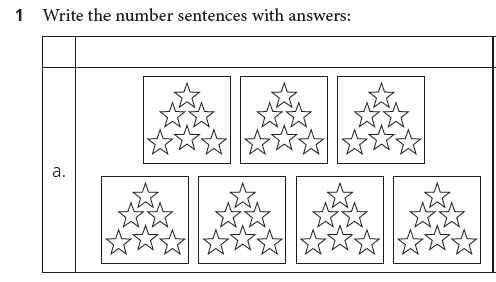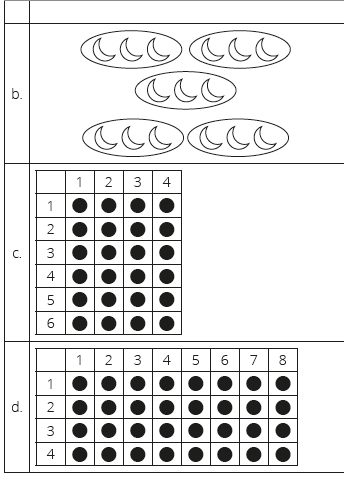1. Calculate by doubling
 Multiple Double Add Answer 1 2 x 16 15 + 1 + 15 + 1 30 + 2 2 2 x 41 3 2 x 26 4 2 x 52 5 2 x 38